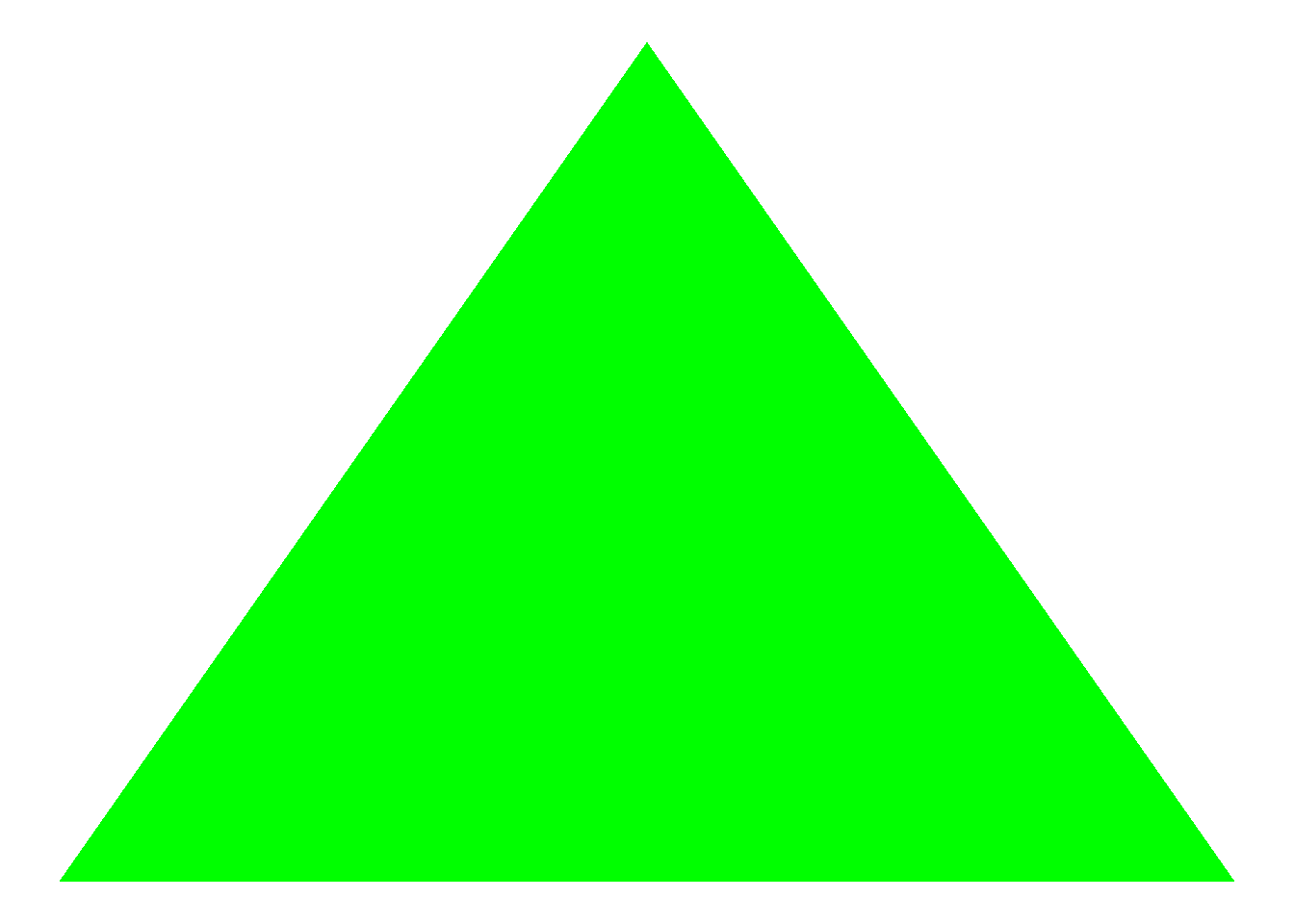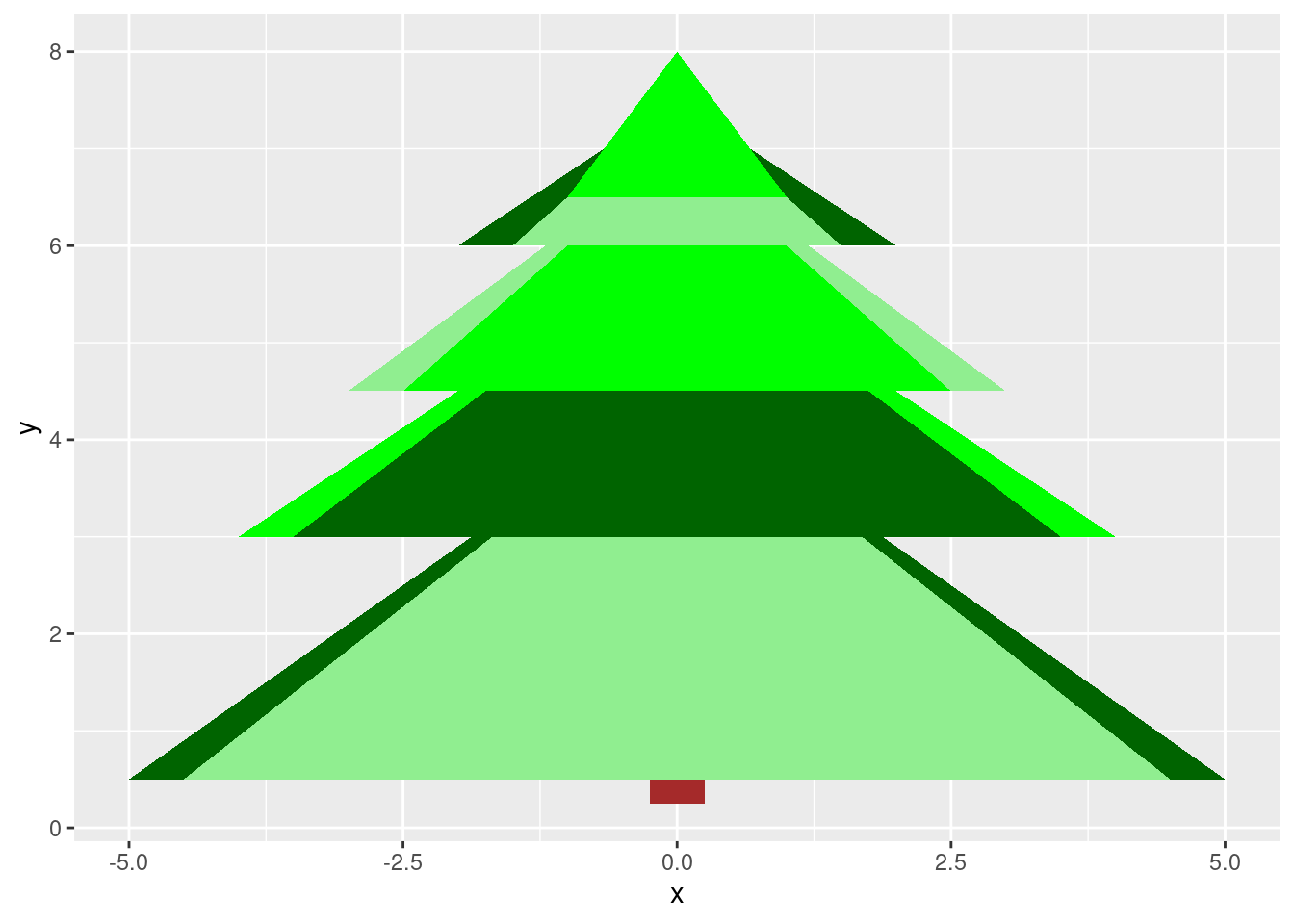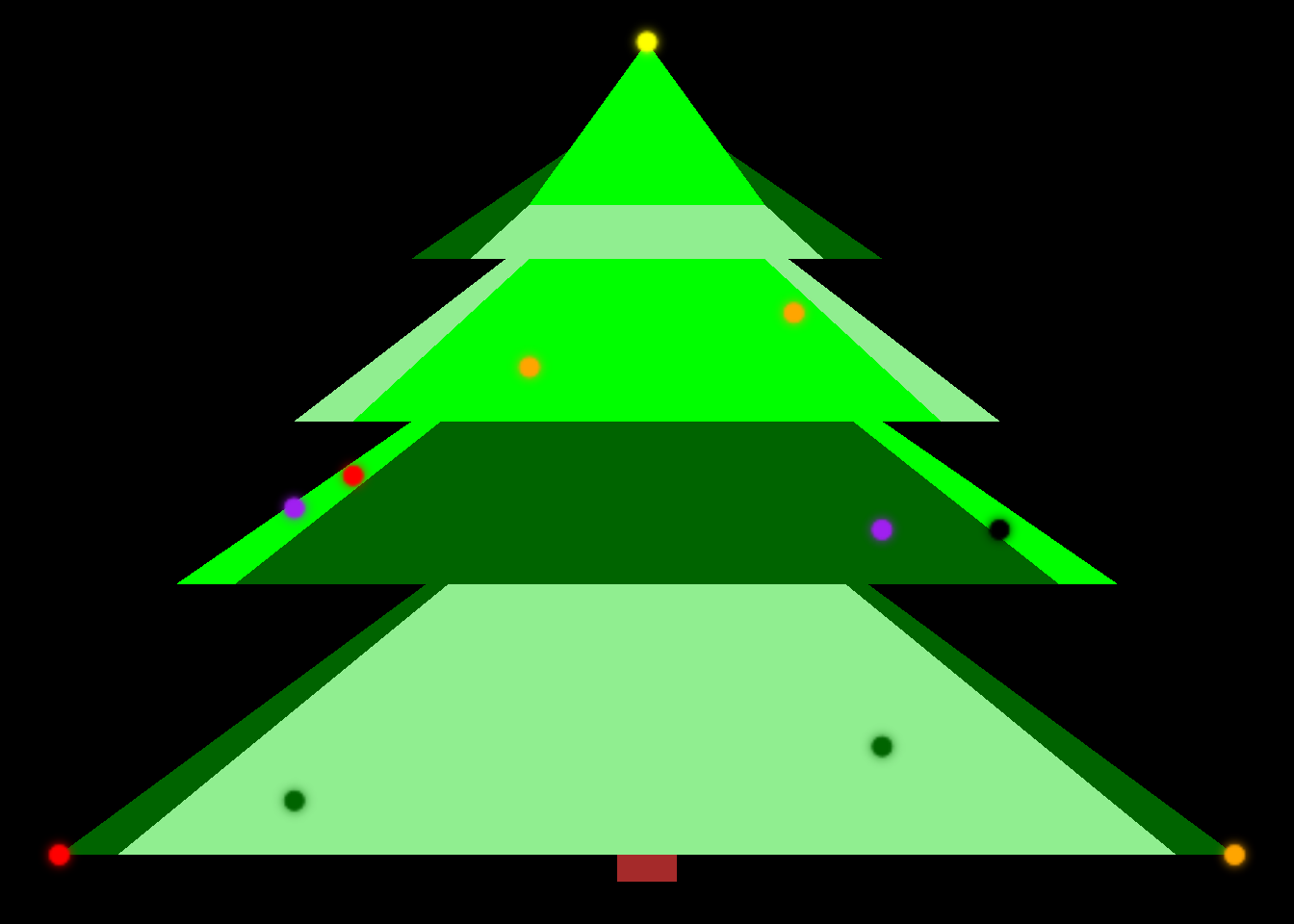# Christmas tree

So it is Christmas and I want a tree. I found this new package experimental ggfx and I thought it would be nice to use it for the lights. Then some time later I found (through twitter) this other repo about building trees.

So here I am building my own christmas tree!

# The very basics

``devtools::install_github('thomasp85/ggfx')``

We’ll build our tree with triangles:

``````library("ggplot2")
ggplot(data.frame(x = c(-5, 5, 0), y = c(0, 0, 5))) +
geom_polygon(aes(x, y), fill = "green") +
theme_void()``````To make it easier we use some functions for the triangles:

``````iso_triangle <- function(wide, height, xvertix, yvertix) {
y <- c(rep(yvertix-height, 2), yvertix)
x <- c(xvertix+wide/2, xvertix-wide/2, xvertix)
data.frame(x, y)
}

square <- function(length, x_bottom_right, y_bottom_right) {
x <- rep(c(x_bottom_right-length, x_bottom_right), 2)
y <- rep(c(y_bottom_right, y_bottom_right+length), each = 2)
data.frame(x, y)[c(1, 3, 4, 2), ]
}``````

And off to build a tree:

``````tree <- ggplot() +
aes(x, y) +
geom_polygon(data = square(0.5, 0.25, 0.25), fill = "brown") +
geom_polygon(data = iso_triangle(10, 4, 0, 4.5), fill = "darkgreen") +
geom_polygon(data = iso_triangle(9, 4, 0, 4.5), fill = "lightgreen") +
geom_polygon(data = iso_triangle(8, 3, 0, 6), fill = "green") +
geom_polygon(data = iso_triangle(7, 3, 0, 6), fill = "darkgreen") +
geom_polygon(data = iso_triangle(6, 2.5, 0, 7), fill = "lightgreen") +
geom_polygon(data = iso_triangle(5, 2.5, 0, 7), fill = "green") +
geom_polygon(data = iso_triangle(4, 1.5, 0, 7.5), fill = "darkgreen") +
geom_polygon(data = iso_triangle(3, 1.5, 0, 7.5), fill = "lightgreen") +
geom_polygon(data = iso_triangle(2, 1.5, 0, 8), fill = "green")
tree``````The tree looks good enough, but if you disagree or want some more realistic trees see this challenge. Very funny to play with it (but I didn’t manage to build my tree with it :/). Anyway, now we have the tree and we need to turn them to a glowing tree!!

# Decorating the tree

Now it is time to decorate and turn on the lights with the help of ggfx:

``````library("ggfx")
tree +
with_blur(geom_point(data =data.frame(x = 0, y = 8), size = 3, col = "yellow"), sigma = 2, stack = TRUE) +
with_blur(geom_point(data =data.frame(x = c(2, -3), y = c(1.5, 1)), size = 3, col = "darkgreen"), sigma = 2, stack = TRUE) +
with_blur(geom_point(data =data.frame(x = c(2, -3), y = 2.5+c(1, 1.2)), size = 3, col = "purple"), sigma = 2, stack = TRUE) +
with_blur(geom_point(data =data.frame(x = -5, y = 0.5), size = 3, col = "red"), sigma = 2, stack = TRUE) +
with_blur(geom_point(data =data.frame(x = 5, y = 0.5), size = 3, col = "orange"), sigma = 2, stack = TRUE) +
with_blur(geom_point(data =data.frame(x = -2.5, y = 4), size = 3, col = "red"), sigma = 2, stack = TRUE) +
with_blur(geom_point(data =data.frame(x = 3, y = 3.5), size = 3, col = "black"), sigma = 2, stack = TRUE) +
with_blur(geom_point(data =data.frame(x = c(-1, 1.25), y = c(5, 5.5)), size = 3, col = "orange"), sigma = 2, stack = TRUE) +
theme_void() +
theme(plot.background = element_rect(fill = "black")) +
NULL``````And now we have our Christmas tree decorated!

Happy Christmas!!

### Reproducibility

``````## ─ Session info ───────────────────────────────────────────────────────────────────────────────────────────────────────
##  setting  value
##  version  R version 4.0.1 (2020-06-06)
##  os       Ubuntu 20.04.1 LTS
##  system   x86_64, linux-gnu
##  ui       X11
##  language (EN)
##  collate  en_US.UTF-8
##  ctype    en_US.UTF-8
##  date     2021-01-08
##
## ─ Packages ───────────────────────────────────────────────────────────────────────────────────────────────────────────
##  package     * version    date       lib source
##  assertthat    0.2.1      2019-03-21  CRAN (R 4.0.1)
##  blogdown      0.21.84    2021-01-07  Github (rstudio/blogdown@c4fbb58)
##  bookdown      0.21       2020-10-13  CRAN (R 4.0.1)
##  cli           2.2.0      2020-11-20  CRAN (R 4.0.1)
##  colorspace    2.0-0      2020-11-11  CRAN (R 4.0.1)
##  crayon        1.3.4      2017-09-16  CRAN (R 4.0.1)
##  digest        0.6.27     2020-10-24  CRAN (R 4.0.1)
##  dplyr         1.0.2      2020-08-18  CRAN (R 4.0.1)
##  ellipsis      0.3.1      2020-05-15  CRAN (R 4.0.1)
##  evaluate      0.14       2019-05-28  CRAN (R 4.0.1)
##  fansi         0.4.1      2020-01-08  CRAN (R 4.0.1)
##  farver        2.0.3      2020-01-16  CRAN (R 4.0.1)
##  generics      0.1.0      2020-10-31  CRAN (R 4.0.1)
##  ggfx        * 0.0.0.9000 2020-08-20  Github (thomasp85/ggfx@4345c5e)
##  ggplot2     * 3.3.2      2020-06-19  CRAN (R 4.0.1)
##  glue          1.4.2      2020-08-27  CRAN (R 4.0.1)
##  gtable        0.3.0      2019-03-25  CRAN (R 4.0.1)
##  htmltools     0.5.0      2020-06-16  CRAN (R 4.0.1)
##  knitr         1.30       2020-09-22  CRAN (R 4.0.1)
##  labeling      0.4.2      2020-10-20  CRAN (R 4.0.1)
##  lifecycle     0.2.0      2020-03-06  CRAN (R 4.0.1)
##  magick        2.5.2      2020-11-10  CRAN (R 4.0.1)
##  magrittr      2.0.1      2020-11-17  CRAN (R 4.0.1)
##  munsell       0.5.0      2018-06-12  CRAN (R 4.0.1)
##  pillar        1.4.7      2020-11-20  CRAN (R 4.0.1)
##  pkgconfig     2.0.3      2019-09-22  CRAN (R 4.0.1)
##  purrr         0.3.4      2020-04-17  CRAN (R 4.0.1)
##  R6            2.5.0      2020-10-28  CRAN (R 4.0.1)
##  ragg          0.4.0      2020-10-05  CRAN (R 4.0.1)
##  Rcpp          1.0.5      2020-07-06  CRAN (R 4.0.1)
##  rlang         0.4.10     2020-12-30  CRAN (R 4.0.1)
##  rmarkdown     2.6        2020-12-14  CRAN (R 4.0.1)
##  scales        1.1.1      2020-05-11  CRAN (R 4.0.1)
##  sessioninfo   1.1.1      2018-11-05  CRAN (R 4.0.1)
##  stringi       1.5.3      2020-09-09  CRAN (R 4.0.1)
##  stringr       1.4.0      2019-02-10  CRAN (R 4.0.1)
##  systemfonts   0.3.2      2020-09-29  CRAN (R 4.0.1)
##  textshaping   0.2.1      2020-11-13  CRAN (R 4.0.1)
##  tibble        3.0.4      2020-10-12  CRAN (R 4.0.1)
##  tidyselect    1.1.0      2020-05-11  CRAN (R 4.0.1)
##  vctrs         0.3.6      2020-12-17  CRAN (R 4.0.1)
##  withr         2.3.0      2020-09-22  CRAN (R 4.0.1)
##  xfun          0.20       2021-01-06  CRAN (R 4.0.1)
##  yaml          2.2.1      2020-02-01  CRAN (R 4.0.1)
##
##  /home/lluis/bin/R/4.0.1/lib/R/library``````##### Lluís Revilla Sancho
###### Bioinformatician

Bioinformatician with interests in functional enrichment, data integration and transcriptomics.